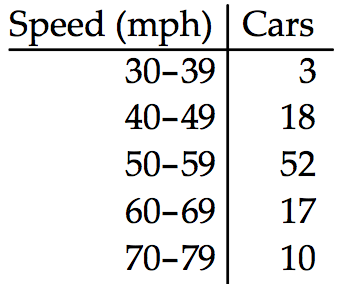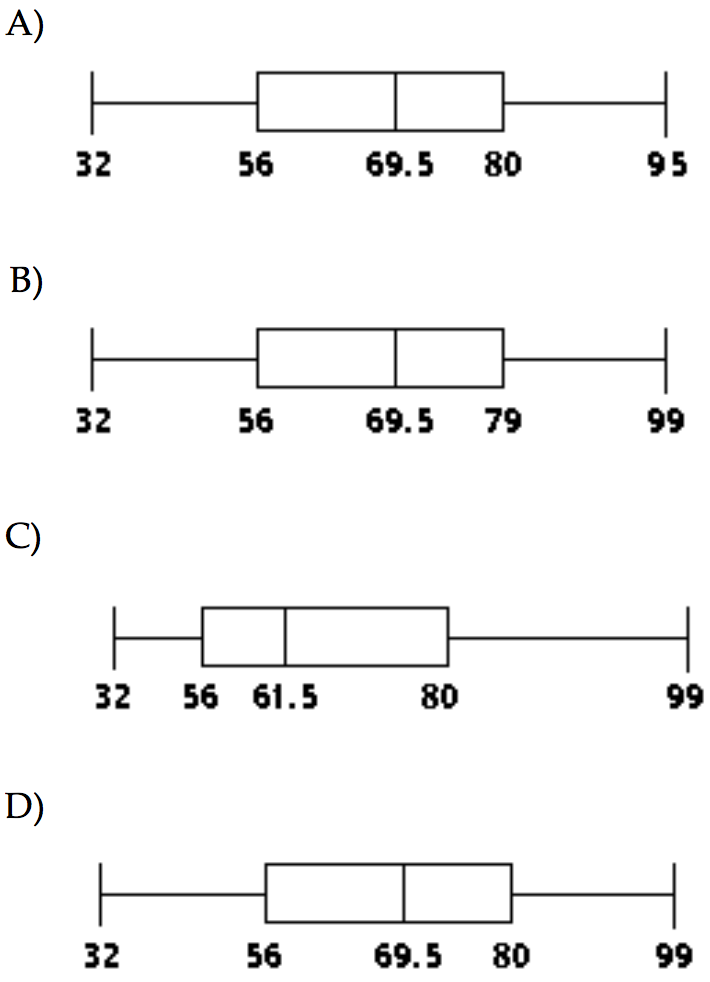Dec 12, 2016

# Describing, Exploring, and Comparing Data — practice test 3B

20 cards
Problems with solutions in Elementary Statistics — Measures of Center, Measures of Variations, Measures of Relative Standing, Exploratory Data Analysis (EDA)

Directions:

• Provide an appropriate response.
• 1. Explain how two data sets could have equal means and modes but still differ greatly. Give an example with two data sets to illustrate.

The sets would have different sizes and standard deviations. Examples will vary. A general method for constructing examples is as follows: (1) compose several values and find the sum; (2) prepare another data set with, say, twice that sum and twice that size. Examples are — Set A: 5, 10, 15, 2, 5, 8; Set B: 9, 10, 11, 3, 5, 7, 19, 1, 2, 5, 6, 12. Notice that Set A and Set B both have means = 7.5 and modes = 5. Yet, the n for A is 6, while the n for B is 12. Also, the s for A is 4.6, while the s for B is 5.1.

1. 2. We want to compare two different groups of students, students taking Composition 1 in a traditional lecture format and students taking Composition 1 in a distance learning format. We know that the mean score on the research paper is 85 for both groups. What additional information would be provided by knowing the standard deviation?

By knowing the standard deviation for both groups, we would have an idea about how the individual scores for each group varied about 85. The smaller standard deviation would indicate that individual scores were closer to 85 than would a larger standard deviation.

1. 3. Describe how to find the percentile for a given score in a set of data. How does this process relate to the definition of a percentile score?

After the data values are arranged from lowest to highest, the number of values lower than the given score should be divided by the total number of values, and this quotient should be multiplied by 100. The percentile for the given score is the nearest whole number to the resulting product. Since a percentile score is a score at a specified percentile, it can be found by the method described above.

2. Find the mean for the given sample data.
• 4. Bill kept track of the number of hours he spent exercising each week. The results for four months are shown below. Find the mean number of hours Bill spent exercising per week. Round your answer to two decimal places.
7.50 8.20 7.10 7.90 8.00 7.50
7.80 7.10 7.30 7.50 7.90 8.90
7.10 8.20 8.20 8.20 8.00 7.80
1. 7.38
2. 8.25
3. 7.79
4. 8.01

3. Find the median for the given sample data.
• 5. A store manager kept track of the number of newspapers sold each week over a seven-week period. The results are shown below:
95, 38, 221, 122, 258, 237, 233.
Find the median number of newspapers sold.
1. 122 newspapers
2. 221 newspapers
3. 233 newspapers
4. 172 newspapers

4. Find the mode(s) for the given sample data.
• 6. 98, 25, 98, 13, 25, 29, 56, 98
1. 55.3
2. 25
3. 98
4. 42.5

5. Find the midrange for the given sample data.
• 7. The weights (in ounces) of 18 cookies are shown. Find the midrange.
0.60 1.34 0.89 0.96 0.80 1.43
1.34 1.16 0.60 1.49 1.34 1.16
1.34 1.49 0.80 1.34 0.96 0.89
1. 1.045
2. 1.16
3. 1.145
4. 1.015

• 8. The highway speeds of 100 cars are summarized in the frequency distribution below. Find the mean speed.1. 54.5 mph
2. 58.6 mph
3. 55.8 mph
4. 61.4 mph

6. Solve the problem.
• 9. Melissa gets quiz grades of 75, 85, and 95. She gets an 80 on her final exam. Find the weighted mean if the quizzes each count for 15% and the final exam counts for 55% of the final grade.
1. 82.1
2. 82.3
3. 82.4
4. 82.2

• 10. The data below consists of the heights (in inches) of 20 randomly selected women. Find the 10% trimmed mean of the data set. The 10% trimmed mean is found by arranging the data in order, deleting the bottom10% of the values and the top 10% of the values and then calculating the mean of the remaining values.
64 62 62 61 67
68 70 67 62 63
61 64 75 67 60
59 64 68 65 71
1. 64.7 in
2. 51.8 in
3. 64.8 in
4. 65.0 in

7. Find the range for the given data.
• 11. Fred, a local mechanic, gathered the following data regarding the price, in dollars, of an oil and filter change at twelve competing service stations:
32.95 24.95 26.95 28.95
18.95 28.95 30.95 22.95
24.95 26.95 29.95 28.95
Compute the range.
1. \$14
2. \$12
3. \$10
4. \$8

• 12. Find the variance for these data. Round your answer to one more decimal place than the original data.
1, 4, –5, –9, and 6
1. 39.3
2. 39.2
3. 39.4
4. 31.4

8. Solve the problem.
• 13. The maximum value of a distribution is 36.3 and the minimum value is 3.3. Use the range rule of thumb to find the standard deviation. Round results to the nearest tenth.
1. 13.3
2. 5.3
3. 8.3
4. 14.9

• 14. The ages of the members of a gym have a mean of 39 years and a standard deviation of 13. Use the range rule of thumb to estimate the minimum and maximum “usual” ages.
1. 13 years, 65 years
2. 12 years, 66 years
3. 15 years, 67 years
4. 26 years, 52 years

9. Use the empirical rule to solve the problem.
• 15. At one college, GPA’s are normally distributed with a mean of 2.7 and a standard deviation of 0.4. What percentage of students at the college have a GPA between 2.3 and 3.1?
1. 95.44%
2. 68.26%
3. 84.13%
4. 99.74%

10. Find the z-score corresponding to the given value and use the z-score to determine whether the value is unusual. Consider a score to be unusual if its z-score is less than -2.00 or greater than 2.00. Round the z-score to the nearest tenth if necessary.
• 16. A body temperature of 99.7F given that human body temperatures have a mean of 98.20F and a standard deviation of 0.62.
1. 2.4; unusual
2. 1.5; not unusual
3. –2.4; unusual
4. 2.4; not unusual

11. Determine which score corresponds to the higher relative position.
• 17. Which is better: a score of 82 on a test with a mean of 70 and a standard deviation of 8, or a score of 82 on a test with a mean of 75 and a standard deviation of 4?
1. the first 82
2. the second 82
3. Both scores have the same relative position.

12. Find the percentile for the data point.
• 18. Data set:
3 11 8 6 3 3 11 6 3 11 2 11 15 4 9 3 12 8 6 11;
data point 6.
1. 40
2. 35
3. 62
4. 25

13. Provide an appropriate response.
• 19. If all the values in a data set are converted to z-scores, the shape of the distribution of the z-scores will be bell-shaped regardless of the distribution of the original data. True or false?
1. False
2. True

14. Construct a boxplot for the given data. Include values of the 5-number summary in all boxplots.
• 20. The test scores of 32 students are listed below. Construct a boxplot for the data set:
32 37 41 44 46 48 53 55
57 57 59 63 65 66 68 69
70 71 74 74 75 77 78 79
81 82 83 86 89 92 95 991. the boxplot D
2. the boxplot C
3. the boxplot A
4. the boxplot B